# Summary¶

The Advance mobile contributions (AMC) mode is a feature set that adds more contributor capabilities to the mobile web experience (project page). This report shows the status of the key performance indicators identified in the Annual Plan as of the end of June 2019.

The feature was was first deployed as an opt-in setting on Arabic, Indonesian, and Spanish Wikipedias on March 20, 2019 due to their relatively large populations of existing mobile editors. On June 17, 2019, the team released a second set of features and included additional Wikipedias for testing and feedback (Italian, Japanese, Persian, and Thai). Please seel the full list of target wikis.

This report reflects the status of the KPIs as of the end of FY18-19 (June 2019). AMC is still in the process of being deployed and has not yet been promoted. Metrics will be re-caculated following full rolllout and promotion in Q1.

## Metrics¶

In the annual plan, the Readers Web team defined the following KPis:

Mobile web edit rate on target wikis.

• Target: 10% increase from last year (overall metric).
• Mobile web edits are defined using the existing edit tag.
• Also reviewed tag edits from the new AMC edits tag (done in T212959) to see if they reach 1/11th of total mobile web edits, defined using the existing edit tag.

Retention rate for opt-in advanced mobile mode amongst medium and high-volume editors (100+ edits previous month) ( eswiki, arwiki, idwiki, thwiki, itwiki, jawiki, fawiki (overall, 100+ edits, 500+ edits).

• Target: At least 60% retention.
• Measured using the opt-in/opt-out button (done in T211197 using the PrepUpdate mf_amc_optin). This is set to true each time someone opts out.

Moderation actions on mobile web on target wikis

• Target: 10% increase from last year - via the logs - not looking at AMC only but overall.
• Moderation actions are defined in T213461

For more links to implementation tasks and technical details, see this overview task T210660

## Mobile Web Edit Rate¶

For this report, we reviewed the mobile edit rate on the following:

• Rate on target wikis where deployed: eswiki, arwiki, idwiki, itwiki, jawiki, fawiki, thwiki
• overall rate across target wikis where AMC has been deployed
• overall rate among active editors, in particular medium and high-volume editors (100+ edits 500+ edits).
• proportion of AMC tagged edits out of the total mobile web edits defined using existing tag.

Notes:

• The tag identifies which edits come from users that have AMC enabled.
• Article editing should be performed in the mobile mode.
• Articles should be tagged advanced mobile edit and mobile web edit when AMC interface enabled
In :
library(IRdisplay)

display_html(
'<script>
code_show=true;
function code_toggle() {
if (code_show){
$(\'div.input\').hide(); } else {$(\'div.input\').show();
}
code_show = !code_show
}
$( document ).ready(code_toggle); </script> <form action="javascript:code_toggle()"> <input type="submit" value="Click here to toggle on/off the raw code."> </form>' )  In : shhh <- function(expr) suppressPackageStartupMessages(suppressWarnings(suppressMessages(expr))) shhh({ library(magrittr); library(zeallot); library(glue); library(tidyverse); library(glue); library(lubridate) library(scales) })  In [ ]: #Collect all mobile web edits along mobile web edits tagged as AMC from all target wikis where AMC was deployed #grouped by user edit count # In terminal # spark2R --master yarn --executor-memory 2G --executor-cores 1 --driver-memory 4G query <- "select date_format(event_timestamp, 'yyyy-MM-dd') as date, wiki_db as wiki, user_edit_count, sum(cast(mobile_web_edit as int)) as mobile_web_edits, sum(cast(amc_edit as int)) as amc_edits from ( select wiki_db, event_timestamp, array_contains(revision_tags, 'mobile web edit') as mobile_web_edit, array_contains(revision_tags, 'advanced mobile edit') as amc_edit, CASE WHEN event_user_revision_count is NULL THEN 'undefined' WHEN event_user_revision_count < 100 THEN 'under 100' WHEN event_user_revision_count >= 100 AND event_user_revision_count < 500 THEN '100-499' ELSE '500+' END AS user_edit_count from wmf.mediawiki_history where event_entity = 'revision' and event_type = 'create' and event_timestamp IS NOT NULL and wiki_db in ('eswiki', 'arwiki', 'idwiki', 'itwiki', 'jawiki', 'fawiki', 'thwiki') and event_timestamp between '2018-06-01' and '2019-06-30' and snapshot = '2019-06' ) edits group by wiki_db, date_format(event_timestamp, 'yyyy-MM-dd'), user_edit_count" results <- collect(sql(query)) save(results, file="R/Data/mobile_web_edit_counts.RData")  In : load("Data/mobile_web_edit_counts.RData") mobile_web_edit_counts <- results  In : mobile_web_edit_counts$date <- as.Date(mobile_web_edit_counts$date, format = "%Y-%m-%d") mobile_web_edit_counts_clean <- mobile_web_edit_counts %>% gather(edit_type, edit_count, mobile_web_edits:amc_edits) %>% arrange(date)  ### Overall rate across all target wikis¶ In : ##Overall monthly web edit counts and yoy change mobile_web_edit_monthly_overall_yoy <- mobile_web_edit_counts_clean %>% filter(edit_type == 'mobile_web_edits') %>% mutate(date = floor_date(date, "month")) %>% group_by(date) %>% summarise(total_mobile_edits = sum(edit_count)) %>% arrange(date) %>% mutate(yearOverYear= total_mobile_edits/lag(total_mobile_edits,12) -1) mobile_web_edit_monthly_overall_yoy  A tibble: 13 × 3 datetotal_mobile_editsyearOverYear <date><dbl><dbl> 2018-06-01271192 NA 2018-07-01263999 NA 2018-08-01289015 NA 2018-09-01284295 NA 2018-10-01301943 NA 2018-11-01282857 NA 2018-12-01293722 NA 2019-01-01341384 NA 2019-02-01304855 NA 2019-03-01330972 NA 2019-04-01325321 NA 2019-05-01351466 NA 2019-06-013385000.2481932 In : vertical_lines <- as.numeric(as.Date(c("2019-03-20", "2019-06-17")))  In : ##Plot overall mobile edits rate. mobile_web_edit_monthly_overall <- mobile_web_edit_counts_clean %>% filter(edit_type == 'mobile_web_edits') %>% mutate(date = floor_date(date, "month")) %>% group_by(date) %>% summarise(total_mobile_edits = sum(edit_count)) %>% ggplot(aes(x=date, y = total_mobile_edits)) + geom_line(color = 'darkturquoise', size = 1 ) + geom_vline(xintercept = as.numeric(as.Date("2019-03-20")), linetype = "dashed", color = "black") + geom_text(aes(x=as.Date('2019-03-20'), y=3E5, label="AMC deployed on Arabic, Indonesian, and Spanish Wikipedias"), size=3.5, vjust = -1.2, angle = 90, color = "black") + scale_y_continuous("total mobile web edits per month", labels = polloi::compress) + scale_x_date("Date", labels = date_format("%b %Y"), date_breaks = "1 month") + labs(title = "Monthly mobile web edits \n on all target wikis where AMC was deployed") + ggthemes::theme_tufte(base_size = 12, base_family = "Gill Sans") + theme(axis.text.x=element_text(angle = 45, hjust = 1), plot.title = element_text(hjust = 0.5), panel.grid = element_line("gray70")) mobile_web_edit_monthly_overall ggsave("Figures/mobile_web_edits_overall_monthly.png", mobile_web_edit_monthly_overall, width = 18, height = 9, units = "in", dpi = 150)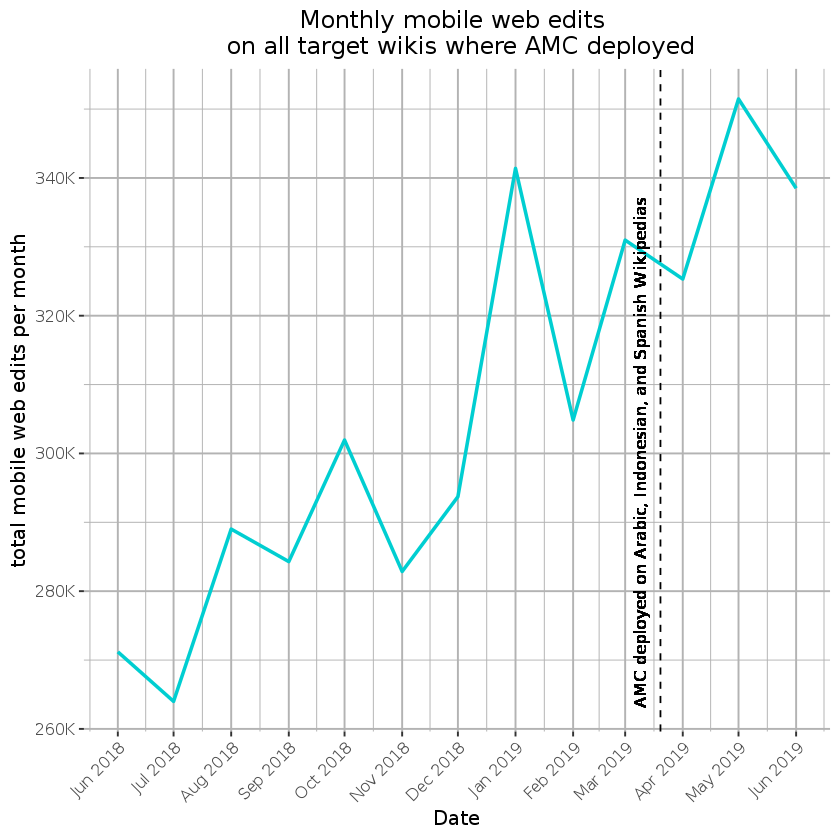In : ##Plot weekl overall mobile edits rate. mobile_web_edit_daily_overall <- mobile_web_edit_counts_clean %>% filter(edit_type == 'mobile_web_edits') %>% #mutate(date = floor_date(date, "month")) %>% group_by(date) %>% summarise(total_mobile_edits = sum(edit_count)) %>% ggplot(aes(x=date, y = total_mobile_edits)) + geom_line(color = 'darkturquoise', size = 1 ) + geom_vline(xintercept = vertical_lines, linetype = "dashed", color = "black") + geom_text(aes(x=as.Date('2019-03-20'), y=8E3, label="AMC deployed on Arabic, Indonesian, and Spanish Wikipedias"), size=3.5, vjust = -1.2, angle = 90, color = "black") + geom_text(aes(x=as.Date('2019-06-17'), y=8E3, label="AMC deployed on Italian, Japanese, Persian, and Thai Wikipedias"), size=3.5, vjust = -1.2, angle = 90, color = "black") + scale_y_continuous("total mobile web edits per day", labels = polloi::compress) + scale_x_date("Date", labels = date_format("%b %Y"), date_breaks = "2 months") + labs(title = "Daily mobile web edits \n on all target wikis where AMC was deployed") + ggthemes::theme_tufte(base_size = 12, base_family = "Gill Sans") + theme(axis.text.x=element_text(angle = 45, hjust = 1), plot.title = element_text(hjust = 0.5), panel.grid = element_line("gray70")) mobile_web_edit_daily_overall ggsave("Figures/mobile_web_edits_overall_daily.png", mobile_web_edit_daily_overall, width = 18, height = 9, units = "in", dpi = 150)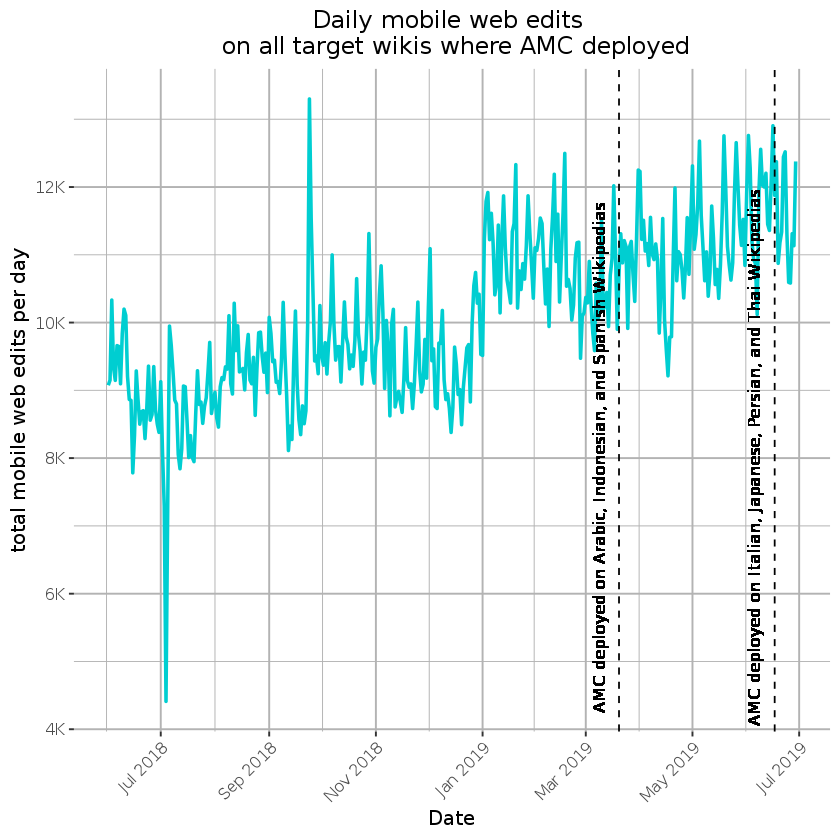There was a steady increase in total mobile web edits the past year, which has been occuring prior to the deployment of AMC on target wikis. This is likely partly due to a sustained increase in overall active editors. AMC was deployed to sets of target wikis on March 20, 2019 and June 17, 2019 but was not yet promoted. We will continue to monitor trends following full deployement and promotion of AMC. ### Mobile web edit rate by edit count¶ In : ## Plot of overall mobile web edit rate by user edit count mobile_web_edit_monthly_byuser <- mobile_web_edit_counts_clean %>% filter(edit_type == 'mobile_web_edits', user_edit_count != 'undefined') %>% ### remove undefined user edit counts mutate(date = floor_date(date, "month")) %>% group_by(date, user_edit_count) %>% summarise(total_mobile_edits = sum(edit_count))%>% ggplot(aes(x=date, y = total_mobile_edits, color = user_edit_count)) + geom_line(size = 1)+ scale_y_continuous("total mobile web edits per month", labels = polloi::compress) + scale_x_date("Date", labels = date_format("%b %Y"), date_breaks = "2 months", limits = c()) + labs(title = "Monthly mobile web edits by user edit count \n on all target wikis where AMC was deployed") + geom_vline(xintercept = vertical_lines, linetype = "dashed", color = "black") + geom_text(aes(x=as.Date('2019-03-20'), y=4.5E4, label="AMC deployed on Arabic, Indonesian, and Spanish Wikipedias"), size=3.5, vjust = -1.2, angle = 90, color = "black") + geom_text(aes(x=as.Date('2019-06-17'), y=4.5E4, label="AMC deployed on Italian, Japanese, Persian, and Thai Wikipedias"), size=3.5, vjust = -1.2, angle = 90, color = "black") + ggthemes::theme_tufte(base_size = 12, base_family = "Gill Sans") + theme(axis.text.x=element_text(angle = 45, hjust = 1), plot.title = element_text(hjust = 0.5), panel.grid = element_line("gray70"), legend.position="bottom") ggsave("Figures/mobile_web_edits_byeditcount.png", mobile_web_edit_monthly_byuser, width = 18, height = 9, units = "in", dpi = 150) mobile_web_edit_monthly_byuser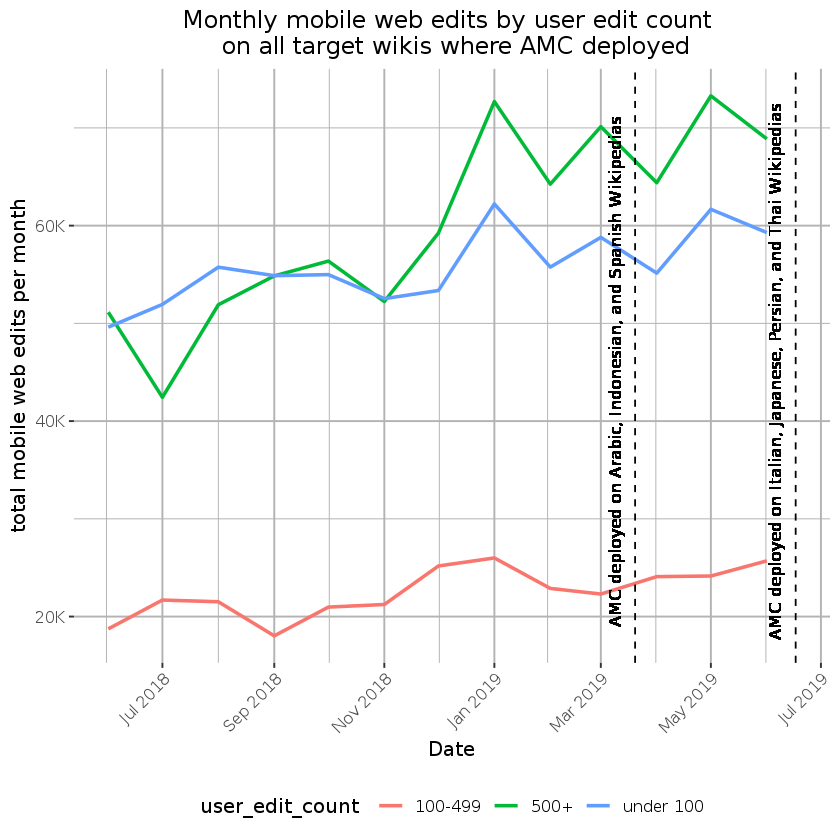In : ##Calculate overall YOY increase for 100+ and 500+ editors across all target wikis mobile_web_edit_under100 <- mobile_web_edit_counts_clean %>% filter(user_edit_count == 'under 100') %>% mutate(date = floor_date(date, "month")) %>% group_by(date) %>% summarise(total_mobile_edits = sum(edit_count)) %>% arrange(date) %>% mutate(yearOverYear= total_mobile_edits/lag(total_mobile_edits,12) -1) mobile_web_edit_100 <- mobile_web_edit_counts_clean %>% filter(user_edit_count == '100-499') %>% mutate(date = floor_date(date, "month")) %>% group_by(date) %>% summarise(total_mobile_edits = sum(edit_count)) %>% arrange(date) %>% mutate(yearOverYear= total_mobile_edits/lag(total_mobile_edits,12) -1) mobile_web_edit_500 <- mobile_web_edit_counts_clean %>% filter(user_edit_count == '500+') %>% mutate(date = floor_date(date, "month")) %>% group_by(date) %>% summarise(total_mobile_edits = sum(edit_count)) %>% arrange(date) %>% mutate(yearOverYear= total_mobile_edits/lag(total_mobile_edits,12) -1)  In : # yoy table edit_count <- c('under 100', '100+', '500+') mobile_web_editcount_yoy <- rbind(mobile_web_edit_under100[13,], mobile_web_edit_100[13,], mobile_web_edit_500[13,]) mobile_web_editcount_yoy$edit_count= edit_count

mobile_web_editcount_yoy

A tibble: 3 × 4
datetotal_mobile_editsyearOverYearedit_count
<date><dbl><dbl><chr>
2019-06-01605580.2205583under 100
2019-06-01262270.3994451100+
2019-06-01700640.3700162500+

There was an increase in the total mobile web edits made by active editors the past year. The largest YoY increae was for the 100-500 editor group.

### Mobile web edit rate by target wiki¶

In :
##Plot of mobile web edits by target wiki.

mobile_web_edit_monthly_bywiki <- mobile_web_edit_counts_clean %>%
filter(edit_type == 'mobile_web_edits',
wiki %in% c('eswiki', 'arwiki', 'idwiki', 'itwiki', 'jawiki', 'fawiki', 'thwiki'))%>%
mutate(date = floor_date(date, "month")) %>%
group_by(date, wiki)%>%
summarise(monthly_edits = sum(edit_count)) %>%
ggplot(aes(x=date, y = monthly_edits, color = wiki)) +
geom_line(size = 1) +
geom_vline(xintercept = vertical_lines,
linetype = "dashed", color = "black") +
geom_text(aes(x=as.Date('2019-03-20'), y=6E4, label="AMC deployed on Arabic, Indonesian, and Spanish Wikipedias"), size=3.5, vjust = -1.2, angle = 90, color = "black") +
geom_text(aes(x=as.Date('2019-06-17'), y=6E4, label="AMC deployed on Italian, Japanese, Persian, and Thai Wikipedias"), size=3.5, vjust = -1.2, angle = 90, color = "black") +
scale_y_continuous("mobile web edits per month", labels = polloi::compress) +
scale_x_date("Date", labels = date_format("%b %Y"), date_breaks = "2 months", limits = c()) +
labs(title = "Monthly mobile web edits on target wikis where AMC was deployed") +
ggthemes::theme_tufte(base_size = 10, base_family = "Gill Sans") +
theme(axis.text.x=element_text(angle = 45, hjust = 1),
plot.title = element_text(hjust = 0.5),
panel.grid = element_line("gray70"),
legend.position= "bottom",
legend.text=element_text(size = 12))

mobile_web_edit_monthly_bywiki

ggsave("Figures/mobile_web_edits_bywiki.png", mobile_web_edit_monthly_bywiki, width = 18, height = 9, units = "in", dpi = 150)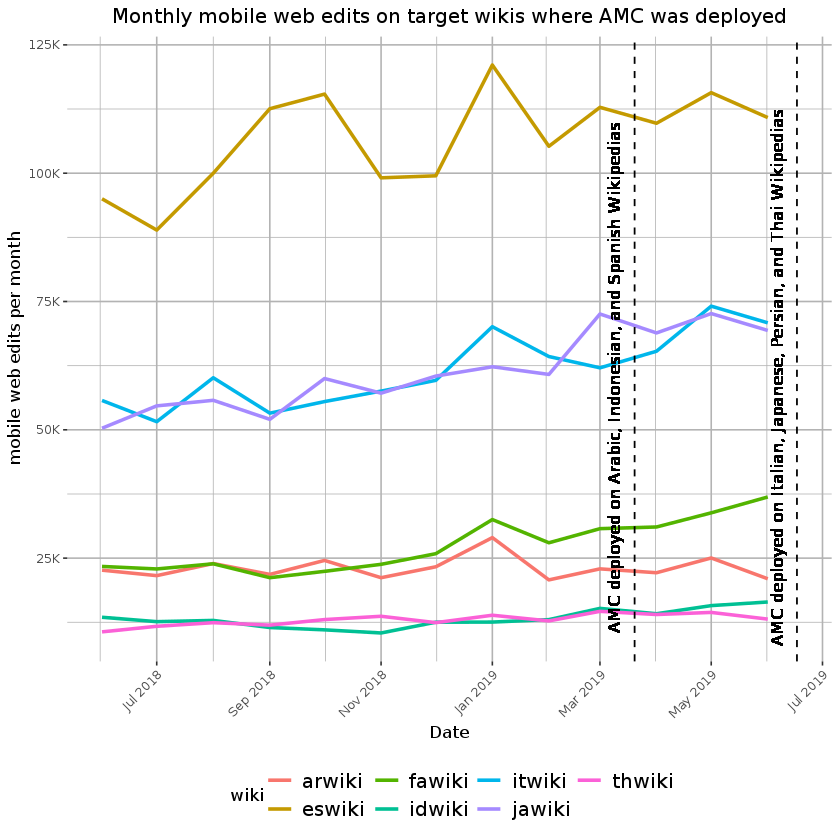In :
##Calculate YOY change for target wikis
#Arwiki
mobile_web_edit_arwiki <- mobile_web_edit_counts_clean %>%
filter(wiki == 'arwiki',
edit_type == 'mobile_web_edits') %>%
mutate(date = floor_date(date, "month")) %>%
group_by(date) %>%
summarise(total_mobile_edits = sum(edit_count)) %>%
arrange(date) %>%
mutate(yearOverYear= total_mobile_edits/lag(total_mobile_edits,12) -1)

#EsWiki
mobile_web_edit_eswiki <- mobile_web_edit_counts_clean %>%
filter(wiki == 'eswiki',
edit_type == 'mobile_web_edits') %>%
mutate(date = floor_date(date, "month")) %>%
group_by(date) %>%
summarise(total_mobile_edits = sum(edit_count)) %>%
arrange(date) %>%
mutate(yearOverYear= total_mobile_edits/lag(total_mobile_edits,12) -1)

#idwiki
mobile_web_edit_idwiki <- mobile_web_edit_counts_clean %>%
filter(wiki == 'idwiki',
edit_type == 'mobile_web_edits') %>%
mutate(date = floor_date(date, "month")) %>%
group_by(date) %>%
summarise(total_mobile_edits = sum(edit_count)) %>%
arrange(date) %>%
mutate(yearOverYear= total_mobile_edits/lag(total_mobile_edits,12) -1)

#itwiki
mobile_web_edit_itwiki <- mobile_web_edit_counts_clean %>%
filter(wiki == 'itwiki',
edit_type == 'mobile_web_edits') %>%
mutate(date = floor_date(date, "month")) %>%
group_by(date) %>%
summarise(total_mobile_edits = sum(edit_count)) %>%
arrange(date) %>%
mutate(yearOverYear= total_mobile_edits/lag(total_mobile_edits,12) -1)

#jawiki
mobile_web_edit_jawiki <- mobile_web_edit_counts_clean %>%
filter(wiki == 'jawiki',
edit_type == 'mobile_web_edits') %>%
mutate(date = floor_date(date, "month")) %>%
group_by(date) %>%
summarise(total_mobile_edits = sum(edit_count)) %>%
arrange(date) %>%
mutate(yearOverYear= total_mobile_edits/lag(total_mobile_edits,12) -1)

#fawiki
mobile_web_edit_fawiki <- mobile_web_edit_counts_clean %>%
filter(wiki == 'fawiki',
edit_type == 'mobile_web_edits') %>%
mutate(date = floor_date(date, "month")) %>%
group_by(date) %>%
summarise(total_mobile_edits = sum(edit_count)) %>%
arrange(date) %>%
mutate(yearOverYear= total_mobile_edits/lag(total_mobile_edits,12) -1)

#thwiki
mobile_web_edit_thwiki <- mobile_web_edit_counts_clean %>%
filter(wiki == 'thwiki',
edit_type == 'mobile_web_edits') %>%
mutate(date = floor_date(date, "month")) %>%
group_by(date) %>%
summarise(total_mobile_edits = sum(edit_count)) %>%
arrange(date) %>%
mutate(yearOverYear= total_mobile_edits/lag(total_mobile_edits,12) -1)

In :
# Create YoY Table
wiki_list <- c('arwiki', 'eswiki', 'idwiki', 'itwiki', 'jawiki', 'fawiki', 'thwiki')
mobile_web_edit_yoy <- rbind(mobile_web_edit_arwiki[13,], mobile_web_edit_eswiki[13,],
mobile_web_edit_idwiki[13,], mobile_web_edit_itwiki[13,],
mobile_web_edit_jawiki[13,], mobile_web_edit_fawiki[13,], mobile_web_edit_thwiki[13,])



### Overall Retention Rate of opt-in AMC¶

In :
##Look at Overall Retention Rate
amc_retention_overall_daily <- amc_retention_rates %>%
filter(date <= '2019-06-30') %>% #filter out July due to incomplete history in mediawikihistory table
#mutate(date = floor_date(date, "week")) %>%
group_by(date, amc_selection) %>%
summarise(n_opt = sum(n_opt)) %>%
ggplot(aes(x=date, y = n_opt, color = amc_selection)) +
geom_line(size = 1)+
scale_y_continuous("Daily count", labels = polloi::compress) +
scale_x_date("Date", labels = date_format("%b %Y"), date_breaks = "2 weeks") +
labs(title = "Daily retention rate of opt-in AMC on all target wikis where deployed") +
geom_vline(xintercept = vertical_lines,
linetype = "dashed", color = "black") +
geom_text(aes(x=as.Date('2019-03-20'), y=30, label="AMC deployed on Arabic, Indonesian, and Spanish Wikipedias"), size=3.5, vjust = -1.2, angle = 90, color = "black") +
geom_text(aes(x=as.Date('2019-06-17'), y= 30, label="AMC deployed on Italian, Japanese, Persian, and Thai Wikipedias"), size=3.5, vjust = -1.2, angle = 90, color = "black") +
ggthemes::theme_tufte(base_size = 12, base_family = "Gill Sans") +
theme(axis.text.x=element_text(angle = 45, hjust = 1),
plot.title = element_text(hjust = 0.5),
panel.grid = element_line("gray70"),
legend.position="bottom")

ggsave("Figures/amc_retention_daily.png", amc_retention_overall_daily, width = 18, height = 9, units = "in", dpi = 150)
amc_retention_overall_daily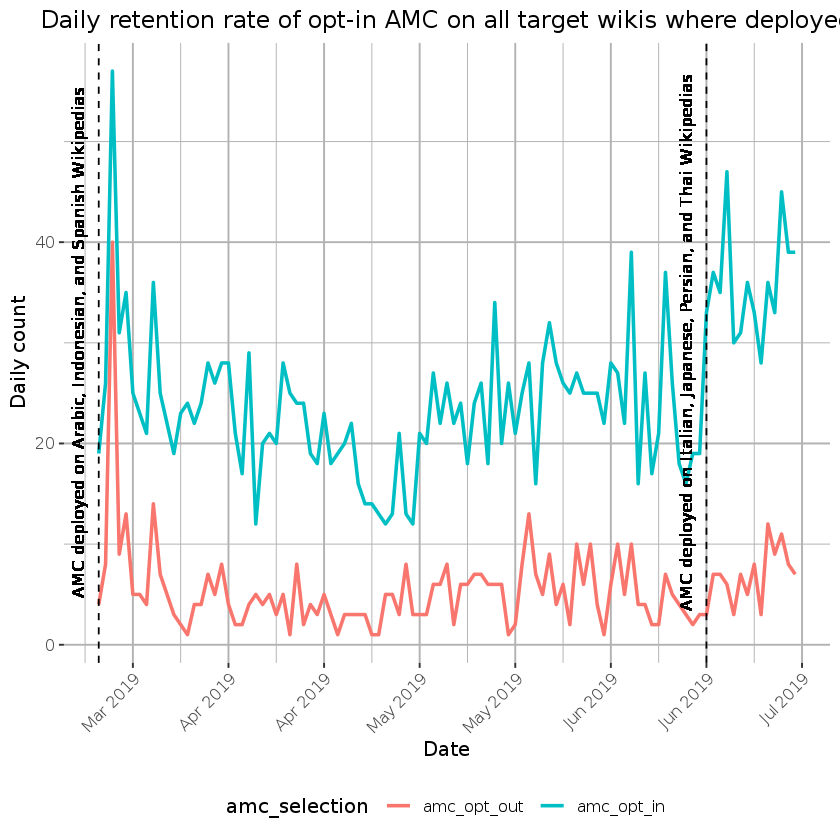There was an 81% overall retention rate of the opt-in AMC mode across all target wikis where AMC was deployed (time period: March 20, 2019-June 30, 2019), surpassing the target of 60%.

### Retention Rate of opt-in AMC by Target Wiki¶

In :
# Overall retention rate

amc_retention_overall_percent <- amc_retention_rates %>%
filter(date <= '2019-06-30') %>% #filter out July due to incomplete history in mediawikihistory table
group_by(wiki, amc_selection) %>%
summarise(n_opt = sum(n_opt)) %>%
group_by(wiki) %>%
mutate(prop_opt_in_percent = amc_opt_in/(amc_opt_out+amc_opt_in)*100)


A grouped_df: 6 × 4
wikiamc_opt_outamc_opt_inprop_opt_in_percent
<chr><dbl><dbl><dbl>
arwiki233119283.64912
eswiki206 72377.82562
fawiki 12 11990.83969
idwiki 92 42082.03125
itwiki 5 3988.63636
jawiki 17 4572.58065
In :
##Overall proportion of AMC retention rates on each target wiki

amc_retention_props <- amc_retention_rates %>%
filter(date <= '2019-06-30') %>% #filter out July due to incomplete date
group_by(wiki, amc_selection) %>%
summarise(n_opt = sum(n_opt)) %>%
ggplot(aes(x=factor(1), y= n_opt, fill = amc_selection)) +
geom_col(position="fill") +
scale_y_continuous(labels = scales::percent_format()) +
facet_wrap(~wiki, scale = "free_y") +
labs(title = "Retention rate of opt-in AMC on all target wikis where deployed",
fill = "AMC Selection",
x= NULL,
y = "Retention rate of opt-in AMC by user edit count") +
ggthemes::theme_tufte(base_size = 12, base_family = "Gill Sans") +
theme(axis.text.x=element_blank(),
plot.title = element_text(hjust = 0.5),
panel.grid = element_line("gray70"),
legend.position="bottom")
ggsave("Figures/amc_retention_prop_bywiki.png", amc_retention_props, width = 18, height = 9, units = "in", dpi = 150)
amc_retention_props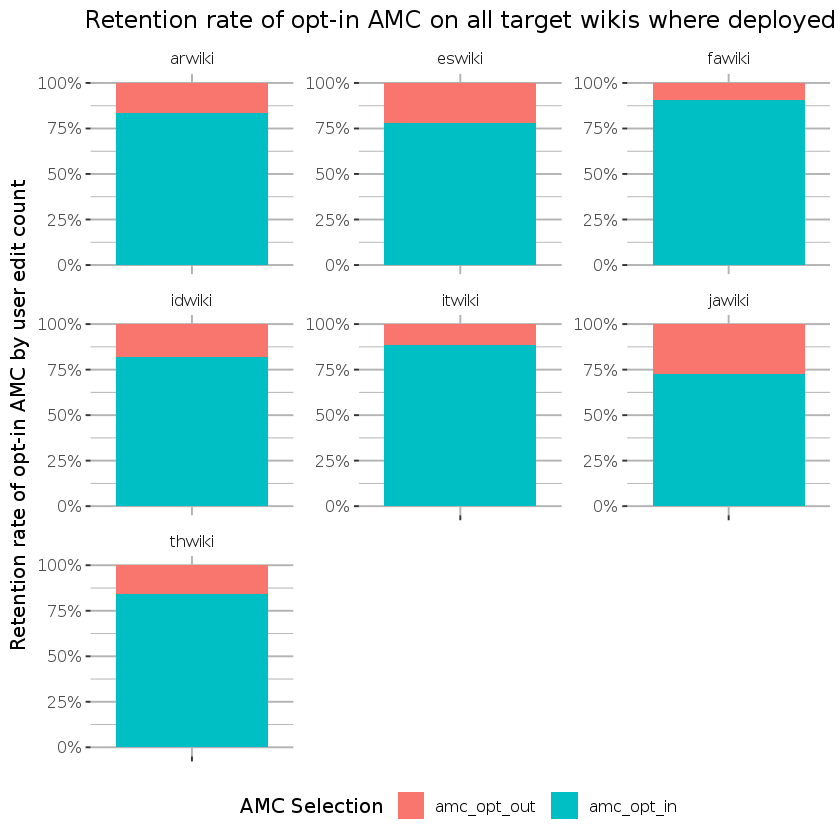On target wikis, opt-in retention rates were high and ranged from 72.6% (Japanese Wikipedia) to 90.8% (Persian Wikipedia).

### Retention Rate of opt-in AMC by user edit count¶

In :
### Overall AMC Retention Rate Broken down by 100+ and 500+ editor groups
## TO Maybe change this to change in proportion over time.

amc_retention_overall_byeditcount <- amc_retention_rates %>%
filter(date <= '2019-06-30') %>% #filter out July due to incomplete history in mediawikihistory table
filter(user_edit_count == '100-499'| user_edit_count == '500+') %>%
mutate(date = floor_date(date, "week")) %>%
group_by(date, amc_selection) %>%
summarise(n_opt = sum(n_opt)) %>%
ggplot(aes(x=date, y = n_opt, color = amc_selection)) +
geom_line(size = 1)+
scale_y_continuous("Weekly count", labels = polloi::compress) +
scale_x_date("Date", labels = date_format("%b %Y"), date_breaks = "1 month") +
labs(title = "Weekly retention rate of opt-in AMC on all target wikis where deployed",
subtitle = "Limited to medium to high-volume editors (100+ and 500+ user edit counts)") +
geom_vline(xintercept = vertical_lines,
linetype = "dashed", color = "black") +
geom_text(aes(x=as.Date('2019-03-20'), y=20, label="AMC deployed on Arabic, Indonesian, and Spanish Wikipedias"), size=3.5, vjust = -1.2, angle = 90, color = "black") +
geom_text(aes(x=as.Date('2019-06-17'), y=20, label="AMC deployed on Italian, Japanese, Persian, and Thai Wikipedias"), size=3.5, vjust = -1.2, angle = 90, color = "black") +
ggthemes::theme_tufte(base_size = 12, base_family = "Gill Sans") +
theme(axis.text.x=element_text(angle = 45, hjust = 1),
panel.grid = element_line("gray70"),
legend.position="bottom")

amc_retention_overall_byeditcount
ggsave("Figures/amc_retention_weekly_byeditcount_.png", amc_retention_overall_byeditcount, width = 18, height = 9, units = "in", dpi = 150)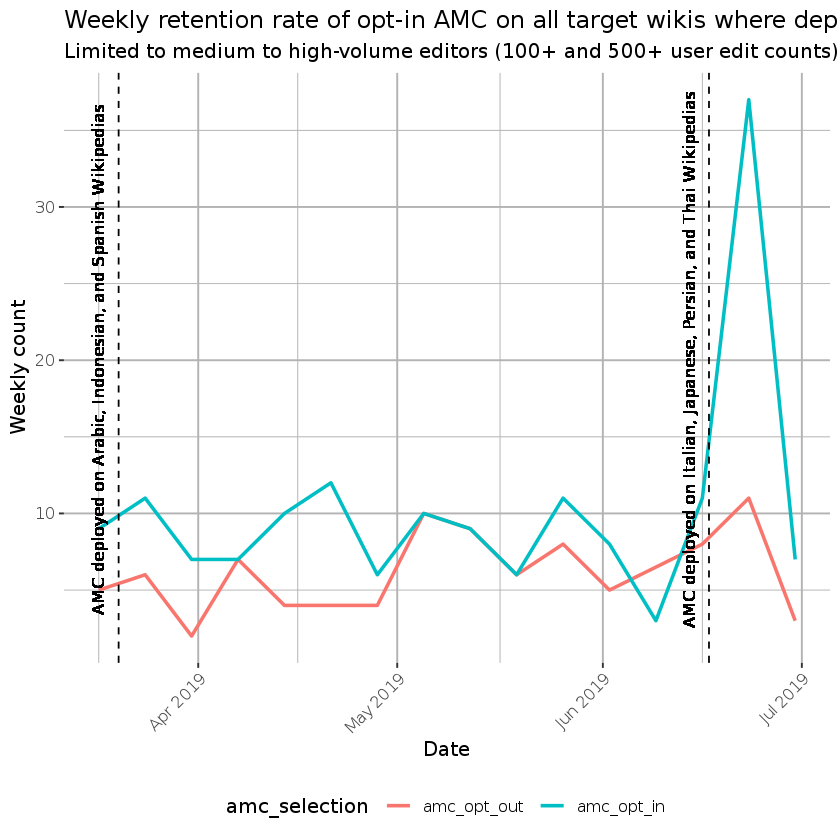In :
# Overall retention rate for 100+ and 500+ editors

amc_retention_prop_overall_byeditor <- amc_retention_rates %>%
filter(date <= '2019-06-30',
user_edit_count == '100-499' | user_edit_count == '500+') %>% #Filter to key user groups and remove incomplete July data
group_by(user_edit_count, amc_selection) %>%
summarise(n_opt = sum(n_opt)) %>%
group_by(user_edit_count) %>%
mutate(prop_opt_in_perct = amc_opt_in/(amc_opt_out+amc_opt_in)*100)

amc_retention_prop_overall_byeditor

A grouped_df: 2 × 4
user_edit_countamc_opt_outamc_opt_inprop_opt_in_perct
<chr><dbl><dbl><dbl>
100-49921 5170.83333
500+ 7111361.41304
In :
##Overall retention rate proportion among 100+ and 500+ editors

amc_retention_props_byeditor <- amc_retention_rates %>%
filter(date <= '2019-06-30',
user_edit_count == 'under 100' | user_edit_count == '100-499' | user_edit_count == '500+') %>% #Filter to remove undefined and incomplete July data
group_by(user_edit_count, amc_selection) %>%
summarise(n_opt = sum(n_opt))%>%
ungroup()%>%
ggplot(aes(x=factor(1), y= n_opt, fill = amc_selection)) +
geom_col(position="fill") +
scale_y_continuous(labels = scales::percent_format()) +
facet_wrap(~ user_edit_count, scale = "free_y") +
labs(title = "Retention rate of opt-in AMC by user edit count",
fill = "AMC Selection",
x = NULL,
y = "Proportion of AMC opt-ins") +
ggthemes::theme_tufte(base_size = 12, base_family = "Gill Sans") +
theme(axis.text.x=element_blank(),
plot.title = element_text(hjust = 0.5),
panel.grid = element_line("gray70"),
legend.position="bottom")

ggsave("Figures/amc_retention_byeditcount.png", amc_retention_props_byeditor, width = 18, height = 9, units = "in", dpi = 150)
amc_retention_props_byeditor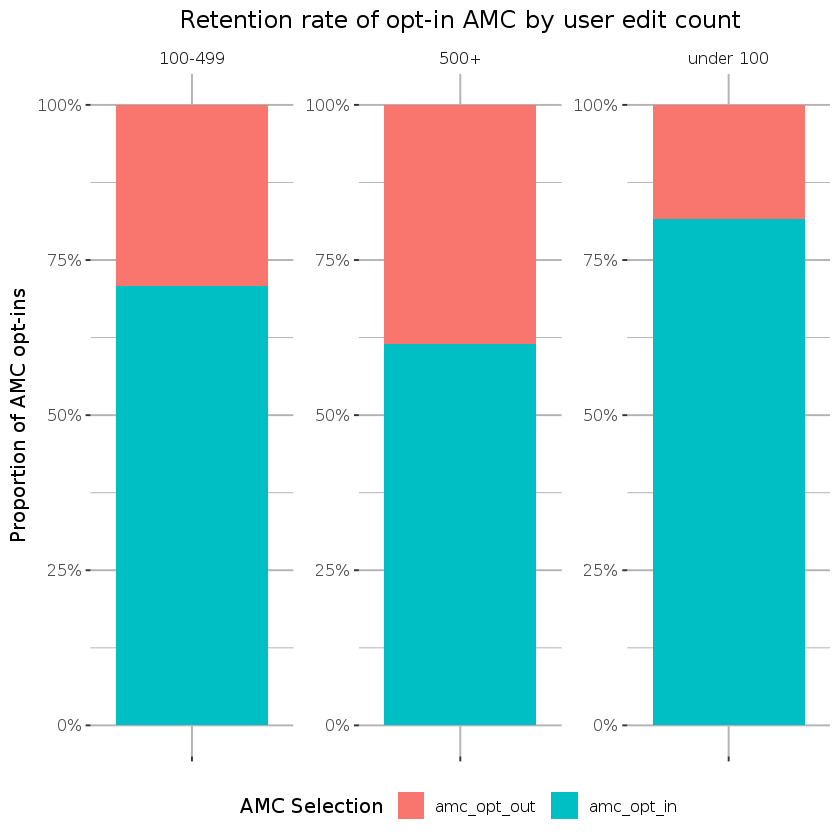The retention rate of AMC was lower for medium to high volume editors compared to low volume editors. There was a 70.8% overall retention rate limited to users with 100+ and 61.4% rate limited to users with 500+ edits (time period: March 20, 2019-June 30, 2019).

## Moderation Actions on Mobile Web¶

We reviewed the rate of moderation actions performed on mobile web on all the target wikis were deployed. The target was a 10% increase from last year on all mobile web (not just AMC tagged edits). Since moderation actions recorded in the log table were not tagged with both the mobile web edit and amc tag until March 18, 2019 (https://phabricator.wikimedia.org/T215477#5008003), we could not calculate a yoy increase for these actions but reviewed changes since March 2019 to June 2019.

Moderation actions are defined in T213461

Final moderation action list:

Using the ct_tag table

• undo (under ct tags tag_name = 'mw-undo'; should show up in user contributions)
• rollback (under ct tags tag_name = 'mw-rollback'; should show up in user contributions)

Using thelogs table

• blocking users (log_action = 'block', from log table)
• unblocking (log_action = 'unblock', from log table)
• deleting (log_action = 'delete', from log table)
• protecting pages (log_action = 'protect', from log table)
• moving pages (log_action = 'move', from log table)
• thanks (log_action = 'thank')
• approving pending changes. Note that (per the documentation, this feature is only available on three of the wikis from the initial AMC deployment, namely arwiki, fawiki idwiki.

Notes:

• When the following actions are executed from an AMC opted-in account, they should be tagged with both advanced mobile edit and mobile web edit in the corresponding public log.
• This analysis currently just includes the increase in moderation actions across all target wiki on mobile web and is not limited to actions tagged as AMC.

TODOs:

• (enwiki-specific:) reviewing new pages (cf. Special:NewPagesFeed)
• other reverts (that are not done via either undo or rollback)
• Investigate mobile web edit tagging to see why delete, unblock and protect are not being tagged correctly. Previous subtask to create and vet query T220148
• Look at proportions of mobile web edits tagged with amc tag
In :
target_wikis <- c(
"eswiki" = "Spanish", "jawiki" = "Japanese",
"itwiki" = "Italian", "fawiki" = "Persian", "arwiki" = "Arabic",
"idwiki" = "Indonesian", "thwiki" = "Thai"
)

In :
## Overall rate for logging table actions on mobile web (not limited to AMC tags) since log items tagged
## March 2019-June 2019
query <- "SELECT
DATE(LEFT(logging.log_timestamp, 8)) as date,
SUM(If(logging.log_type = 'block' and logging.log_action = 'block', 1, 0)) as num_block,
SUM(If(logging.log_type = 'block' and logging.log_action = 'unblock', 1, 0)) as num_unblock,
SUM(If(logging.log_type = 'delete' and logging.log_action = 'delete', 1, 0)) as num_delete,
SUM(If(logging.log_type = 'protect' and logging.log_action = 'protect', 1, 0)) as num_protect,
SUM(If(logging.log_type = 'move' and logging.log_action = 'move', 1, 0)) as num_move,
SUM(If(logging.log_type = 'thanks' and logging.log_action = 'thank', 1, 0)) as num_thank,
SUM(If(logging.log_type = 'review' and logging.log_action = 'approve', 1, 0)) as num_approve
FROM logging as logging
INNER JOIN  (
SELECT ct_rev_id as rev_id,
change_tag_def.ctd_name as tag_name,
change_tag.ct_log_id as log_id
FROM change_tag
INNER JOIN change_tag_def ON (
change_tag.ct_tag_id = change_tag_def.ctd_id
)
) as ct
ON logging.log_id = ct.log_id
WHERE logging.log_timestamp >= '20190301' and
logging.log_timestamp < '20190701' and
ct.tag_name like '%mobile edit%' and
(ct.tag_name like '%mobile web edit%' or ct.tag_name not like '%mobile app edit%')
GROUP By DATE(LEFT(log_timestamp, 8))"

In :
moderation_counts_log <- map_df(
set_names(names(target_wikis), names(target_wikis)),
.id = "wiki"
)
save(moderation_counts_log, file="Data/moderation_counts_log.RData")

In :
load("Data/moderation_counts_log.RData")
moderation_counts_log$date <- as.Date(moderation_counts_log$date, format = "%Y-%m-%d")

In :
# Query to collect unblock and rollback from the user table. Done using change tags now available in mediawiki_history

# In terminal
# spark2R --master yarn --executor-memory 2G --executor-cores 1 --driver-memory 4G

query <- "select
date_format(event_timestamp, 'yyyy-MM-dd') as date,
wiki_db as wiki,
sum(cast(mw_rollback as int)) as num_rollback,
sum(cast(mw_undo as int)) as num_undo
from (
select
wiki_db,
event_timestamp,
array_contains(revision_tags, 'mw-rollback') as mw_rollback,
array_contains(revision_tags, 'mw-undo') as mw_undo
from wmf.mediawiki_history
where
event_timestamp IS NOT NULL and
array_contains(revision_tags, 'mobile web edit') and
wiki_db in ('eswiki', 'arwiki', 'idwiki', 'itwiki', 'jawiki', 'fawiki', 'thwiki') and
event_timestamp >= '2019-03-01' and   event_timestamp < '2019-07-01' and
snapshot = '2019-06'
) edits
group by wiki_db, date_format(event_timestamp, 'yyyy-MM-dd')"

results <- collect(sql(query))
save(results, file="R/Data/moderation_counts_ct.RData")

Error in UseMethod("collect"): no applicable method for 'collect' applied to an object of class "c('sql', 'character')"
Traceback:

1. collect(sql(query))
In :
load("Data/moderation_counts_ct.RData")
moderation_counts_ct <- results
moderation_counts_ct$date <- as.Date(moderation_counts_ct$date, format = "%Y-%m-%d")

In :
##Join the two moderation count tables
moderation_counts_all <- inner_join(moderation_counts_log, moderation_counts_ct,
by = c("date", "wiki"))


### Moderation action rate by action type¶

In :
moderation_counts_bytype_total <- moderation_counts_all %>%
gather(action_type, action_count, num_block:num_undo) %>%
filter(date >= '2019-03-18') %>% #date where log actions were tagged
group_by(action_type) %>%
summarise(action_count = sum(action_count)) %>%
ggplot(aes(x=action_type, y= action_count, fill = action_type)) +
geom_bar(stat='identity') +
geom_text(aes(label=action_count), vjust=0) +
labs(title = "Moderation actions rate on target wikis by action type \n March 2019-June 2019") +
ylab("total counts")+
xlab("type") +
ggthemes::theme_tufte(base_size = 11, base_family = "Gill Sans") +
theme(axis.text.x=element_text(angle = 45, hjust = 1),
plot.title = element_text(hjust = 0.5),
panel.grid = element_line("gray70"),
legend.position = "none")

moderation_counts_bytype_total
ggsave("Figures/moderation_counts_bytype_total.png", moderation_counts_bytype_total, width = 18, height = 9, units = "in", dpi = 150)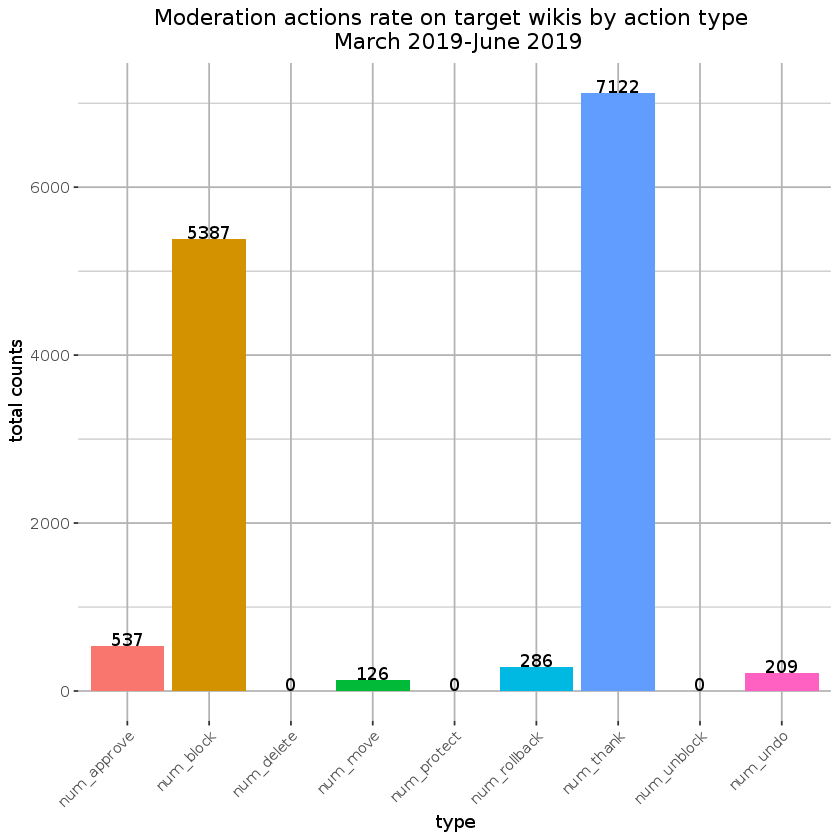In :
#Plot overall moderation actions by action type

moderation_counts_bytype_monthly <- moderation_counts_all %>%
gather(action_type, action_count, num_block:num_undo) %>%
filter(date >= '2019-03-18') %>%
mutate(date = floor_date(date, "month")) %>%
group_by(date, action_type) %>%
summarise(action_count = sum(action_count)) %>%
ggplot(aes(x=date, y = action_count, color = action_type)) +
geom_line(size = 1)+
scale_y_continuous("Monthly action count", labels = polloi::compress) +
scale_x_date("Date", labels = date_format("%b %Y"), date_breaks = "1 month") +
labs(title = "Monthly moderation actions rate on target wikis by action type") +
geom_vline(xintercept = as.Date('2019-03-20'),
linetype = "dashed", color = "black") +
geom_text(aes(x=as.Date('2019-03-20'), y=900, label="AMC deployed on Arabic, Indonesian, and Spanish Wikipedias"), size=3.5, vjust = -1.2, angle = 90, color = "black") +
ggthemes::theme_tufte(base_size = 11, base_family = "Gill Sans") +
theme(axis.text.x=element_text(angle = 45, hjust = 1),
plot.title = element_text(hjust = 0.5),
panel.grid = element_line("gray70"),
legend.position="right")

moderation_counts_bytype_monthly
ggsave("Figures/moderation_counts_bytype_monthly.png", moderation_counts_bytype_monthly , width = 18, height = 9, units = "in", dpi = 150)